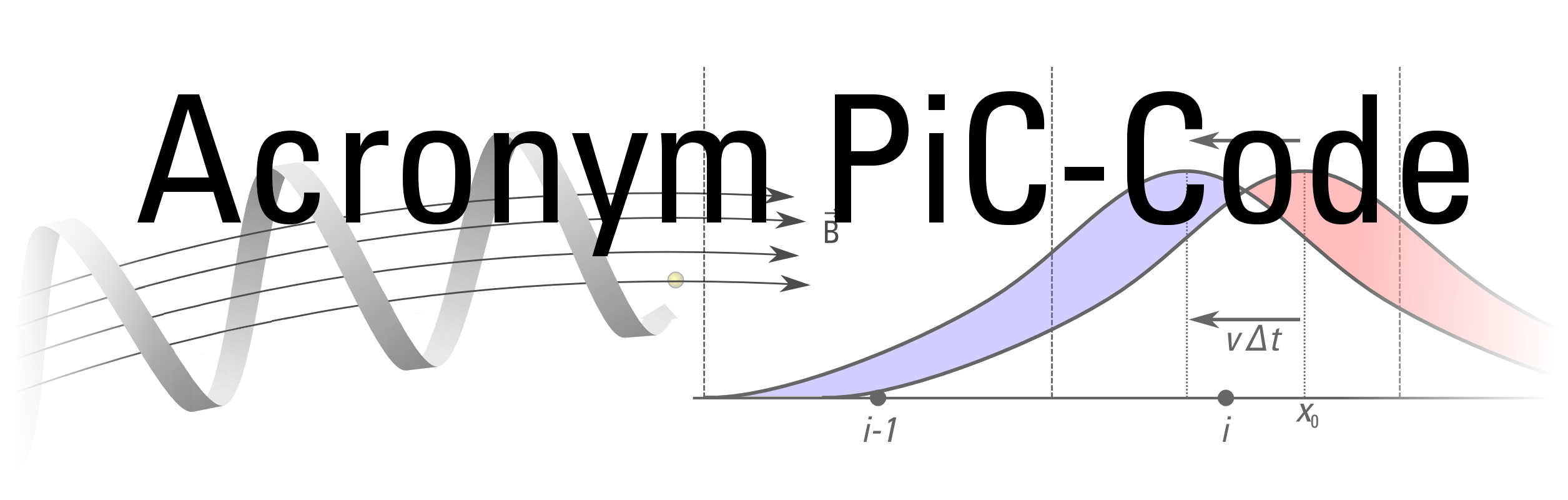# Features

The code is very flexible and can easily be extended to include new setups and algorithms. Some of the implemented features are presented below:

## Particle Push

Particle push is the procedure that updates both the position and velocity of each particle based on the electric and magnetic fields at the location of the particle. Position and velocity are leap-frogged over each other, that is they are defined at half a timestep offset from each other. This makes the scheme second order accurate in time.

### Boris Push

Usually the code uses the original Boris push in the relativistic version as defined in Boris, 1970. (The paper is annoyingly hard to find. It was titled Relativistic plasma simulation - optimization of a hybrid code and was published in the Proceedings of the Fourth Conference on Numerical Simulation Plasmas.)

### Vay Modification

For simulations where particles reach more than mildly relativistic velocities the code also implements the modifications to the Boris push that were proposed by Vay, 2008.

## Staggered Grid

Unlike many Eulerian codes that have all components of the fields that are stored in the grid located at the center of each grid cell, it is common in particle-in-cell codes to have different field components at different locations. This is especially useful for the commonly used electromagnetic plasma model, where the update of the field quantities in time requires the use of the curl of the dual field. The most widely used arrangement, which is also used in ACRONYM, is the so called Yee lattice, as proposed by Yee, 1966. In principle the locations of the electric and magnetic fields can be interchanged without losing the advantageous properties, however we have decided on the layout shown below, as it makes the implementation of perfect electric conductors easier.

### Electric Field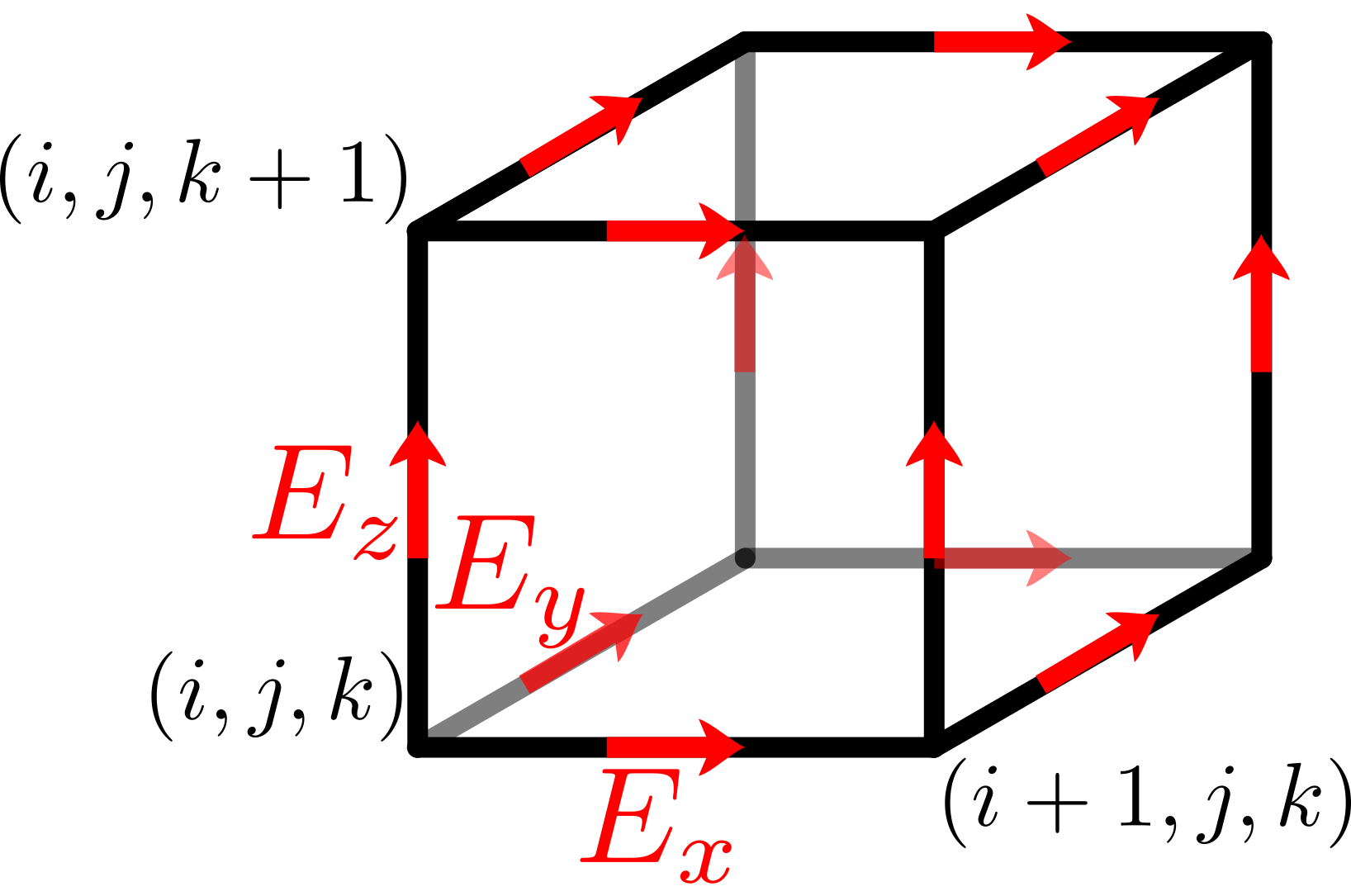The electric field components are located at grid edges, displaced to the center of the edge that is aligned with the direction of the field component. That is the x component E_x of the electric field is displaced by half a grid spacing dx along the edge e_x.
For a perfectly electrically conducting wall at x=0, this arrangement locates the y and z component exactly at the surface of the conductor, where they are trivially forced to zero.

### Magnetic Field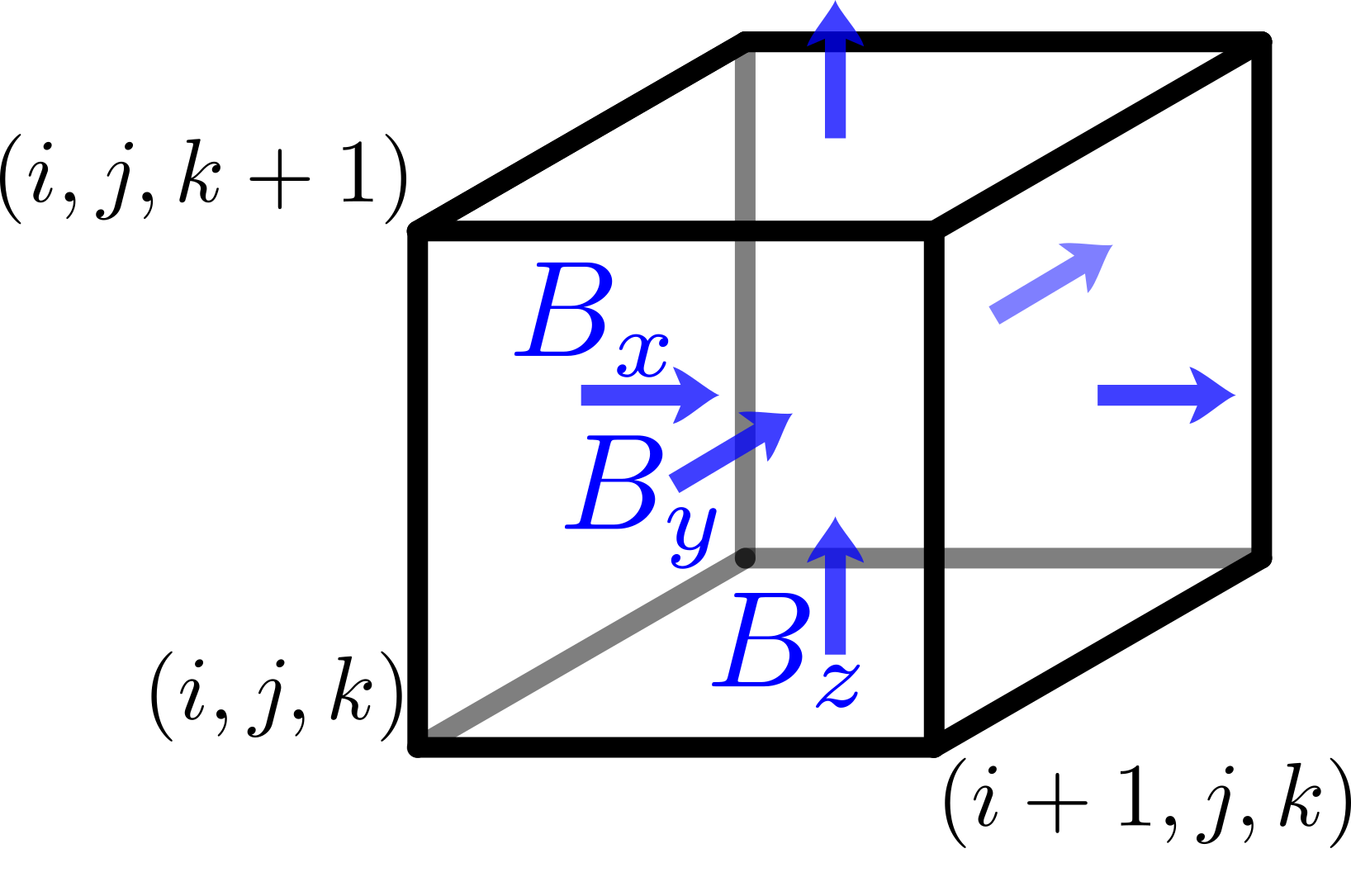The magnetic field components are located on the dual grid, that is on the cell faces. The x component B_x of the magnetic field is located at the center of the face defined by e_y and e_z.
Therefore the electric field components E_y and E_z are located on the cell edges around the magnetic field component B_x, which is optimal for the calculation of curl(E)_x that is needed to update B_x.

### Electric Current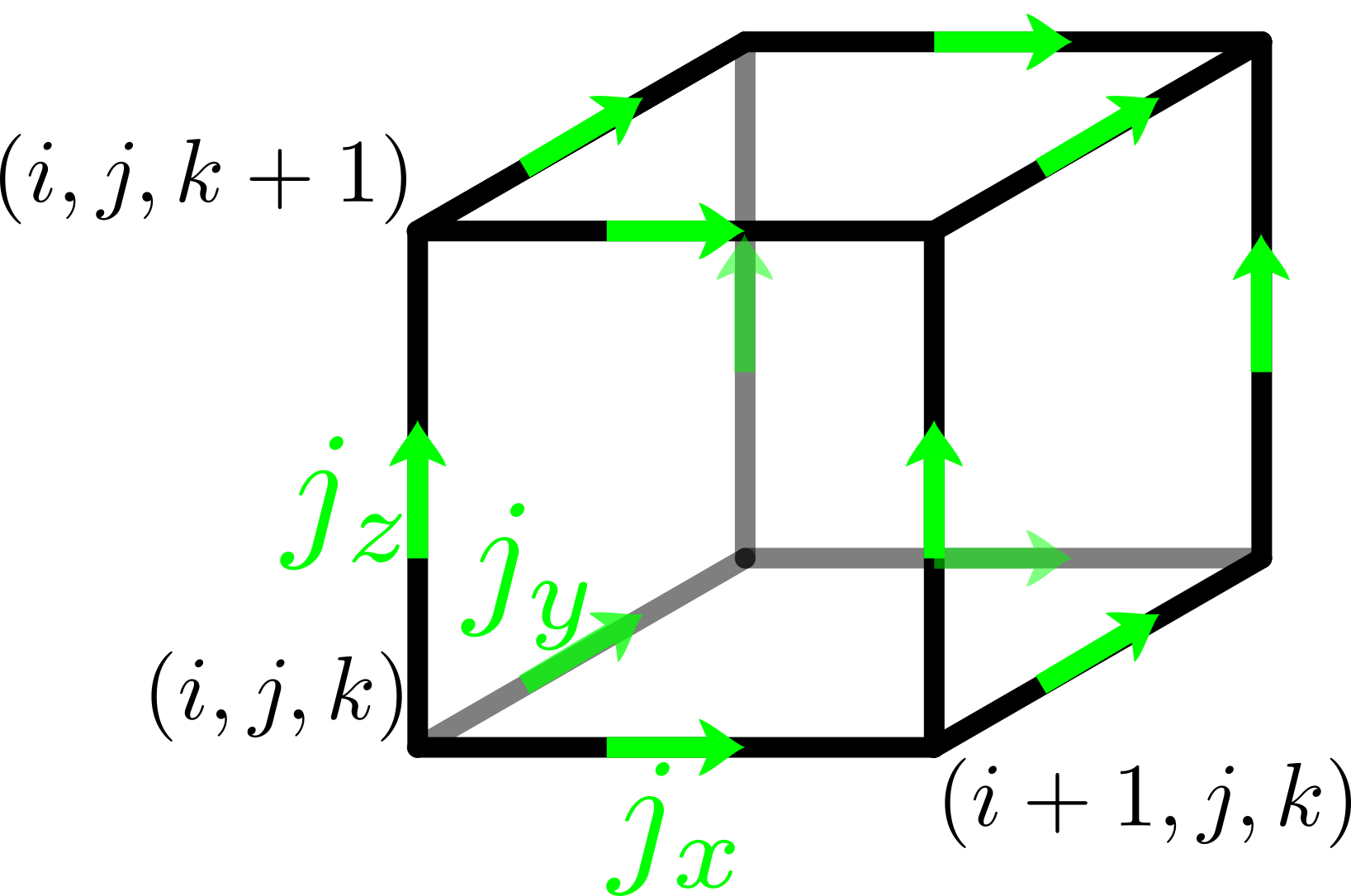The components of the electric current (and mass flow, if computed for analysis purposes) are located at the mid points of cell edges.
In the electromagnetic plasma model the update of a component E_i of the electric field needs the curl curl(B)_i of the magnetic field and the corresponding component j_i of the current. Therefore it is beneficial to collocate the current density with the electric field.

### Charge DensityThe charge density is located in the corner of the grid cell. The center of the grid cell would seem more intuitive, as it would then represent all charges in the cell, but the location in the corner makes the formulation of the Poisson equation (for the electrostatic plasma model) and of the discrete continuity equation (when testing the electromagnetic plasma model) much easier.

## Shape Functions

Particle-in-cell codes are, as the name already implies, a mix of an Eulerian gridded description of the fields and a Langrangian description of freely moving particles. Connecting the two parts requires interpolation between the staggered grid described above and the arbitrary position of a particle. Many different interpolation schemes have been devised over the years. Schemes that decompose into products of one-dimensional shape functions can easily be implemented in ACRONYM and actually a large number of them is supported. The most commonly used schemes of 1st, 2nd, and 3rd order are shown in a little bit more detail below. For additional details we refer to the paper Different Choices of the Form Factor in Particle-in-Cell Simulations by Kilian et al., 2013.

### Cloud in Cell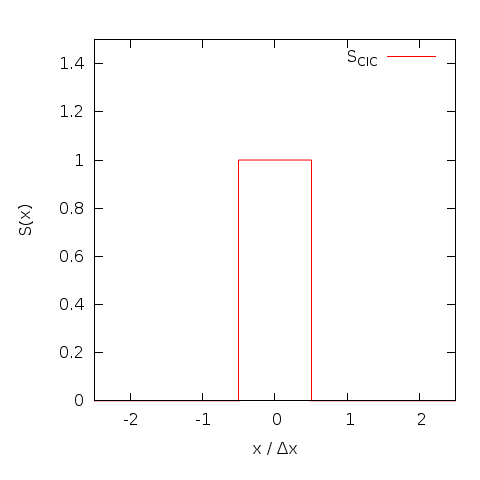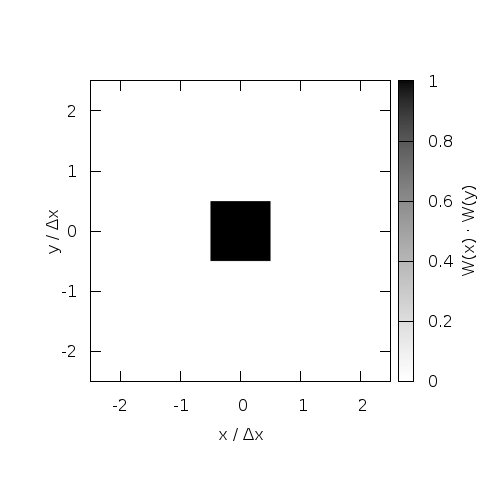While assigning particles simply to the nearest grid point (NGP) is possible, this trivial zeroth order interpolation introduces too much numerical noise to be useful. Assigning a finite size of one grid cell to particles strongly reduces this noise. The overlap integral between the charge cloud representing the particle and the grid cell (area weighting) can be calculated analytically beforehand and the resulting computation is equivalent to a linear interpolation. This CIC method was originally introduced by Birdsall and Fuss, 1969 and made semi-Langrangian plasma simulations feasible.

### Triangular Shaped Cloud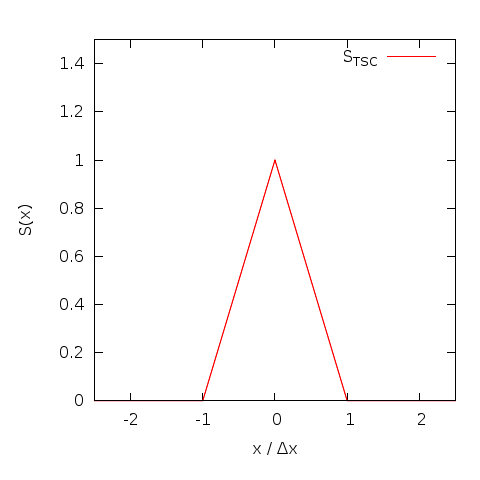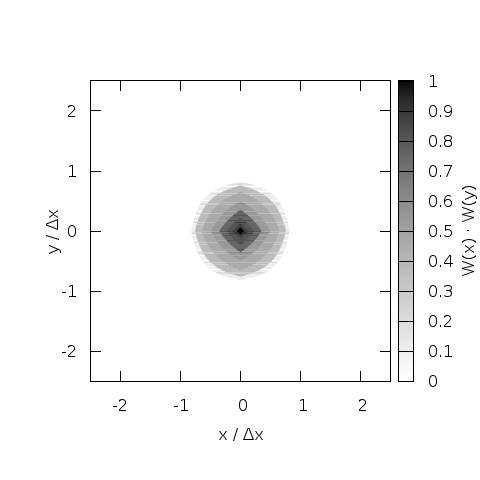While CIC is a big improvement over NGP, it is not perfect. A widely discussed (see e.g. chapter 5 in the standard reference Computer simulations using particles by Hockney and Eastwood, 1988) improvement is the triangular shaped cloud (TSC) that replaces the piecewise defined cloud of CIC with a continuous, piecewise linear function. This increases the effective size of the particle and the number of grid cells involved in the interpolation somewhat, but the reduced noise level and the improved isotropy usually justifies the cost.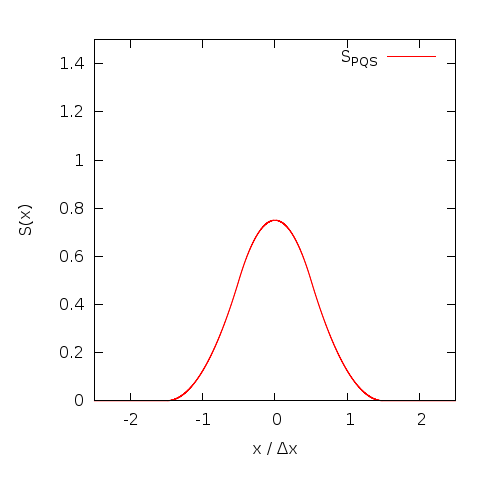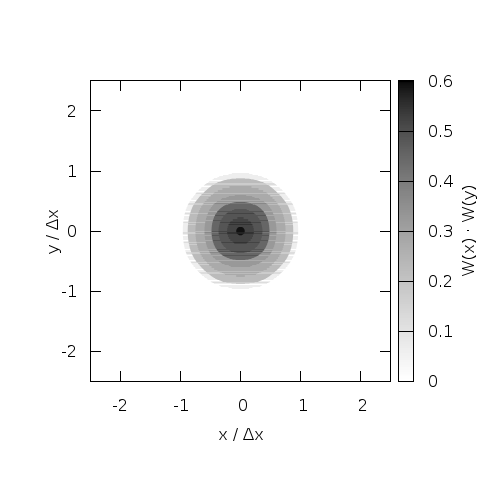The next higher order interpolation function is the piecewise quadratic shape (PQS), which is not only continuous, but also differentiable. This improves roll-off of the Fourier transform by 3dB per octave (a function that has a discontinuity in the nth derivative has a Fourier transform that rolls of proportional to 1/k^{n+1}). If the additional cost due to the growing number of grid cells that are involved in the interpolation is justified depends on the tolerable noise level and the dimensionality of the problem.

## Current Deposition

Charge deposition is relatively straight forward once a shape function is selected. For an electrostatic particle-in-cell code it is sufficient to calculate the electric field and continue. An electromagnetic code on the other hand needs the electric current as a source term in the Maxwell solver. Naive deposition (interpolating q*v at the position of the particle using the shape function directly) leads to a current distribution that does not satisfy the discrete continuity equation. This in turn leads to ghost charges, violation of Gauss law and phantom forces. While it is possible to correct the computed current distribution to remove these effects (and the code does support divergence cleaning following the scheme by Marder, 1987) it is also possible to construct current deposition schemes that directly conserve continuity of electric charges.
The code supports two different such schemes (details below). Additionally it can compute and deposit quantities such as mass density, mass flow, number density, and moments of the distribution function, both as a total over all species and separated per species. These quantities are not needed for the temporal evolution, but can be valuable diagnostics when investigating the physics of a problem.

### Esirkepov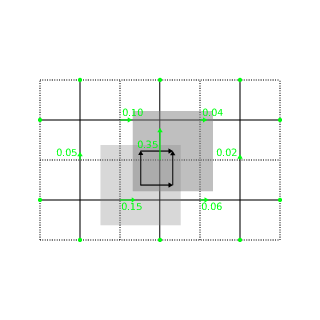The default current deposition scheme follows the method proposed by Esirekepov, 2001. It decomposes the particle motion into axis parallel motions that are weighted in such a way that the correct total current is produced and charge conservation is satisfied. This method works with all shape functions and shows good performance.

### Umeda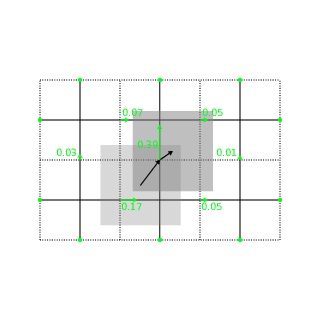Alternatively the code also supports the current deposition algorithm by Umeda, 2003. This algorithm decomposes the particle motion into straight segments with the constraint that the particle can only cross cell boundaries at the middle of cell faces. Only CIC and TSC shape functions are supported. It should be possible to extend this to other shape functions, but to our knowledge this is beyond the published state of the art. Main advantage of the deposition method is a different distribution of numerical noise in k-omega space that might be advantageous to some simulations.

## Field Solver

The ACRONYM code supports a large number of different field solvers, that have different tradeoff between computational cost, numerical noise, and accuracy. They can be roughly divided into three classes: Electrostatic solver, where the electric field is determined by the charge density through Poissons equation; Electromagnetic solver, where both electric and magnetic fields are updated self consistently from the current density; and the radiation free plasma model, which neglects the displacement current and removes electromagnetic waves by construction. Warm collisionless plasma (in excess of 1 MK) is usually best handled by the electromagnetic solvers. However if applicable, the other solvers can speed up the computations by one or two orders of magnitude, as the restrictive CFL condition on the time step due to electromagnetic waves is removed.

### Electrostatic

In the electrostatic plasma model the (longitudinal) electric field is determined by the net charge density of all particles. The Poisson equation that has to be solved is elliptic, i.e. exhibits instantaneous propagation and requires a global field solver. While surprising at first this can be thought of as the limit of long timesteps (much longer than the propagation time of electromagnetic waves through the simulation domain) or the limit of infinite speed of light. Transverse electric fields are not present in this model and the magnetic field is static. This plasma model is only applicable in the absence of large electric current and (equivalently) slow (certainly non-relativistic) particle motion. Depending on the boundary conditions (mixed boundary conditions are not supported in this plasma model) one of two different solvers is chosen.

#### Spectral Solver

If the domain is periodic, the Poisson equation of the electrostatic plasma model is solved using a spectral solver. The code deposits the charge density of all particles to the grid and computes the Fourier transform of it. In Fourier space the Poisson equation can easily be solved for each different k, allowing for the direct construction of of the Fourier transform of (one component of) the electric field. A subsequent inverse Fourier transform produces the electric field in real space. The global Fourier transform over all spatial dimensions is computed using a modified version of the Sandia FFT that allows to use FFTW 3 as the underlying local 1d transformation. Two features that are described in the literature but not used in all codes are the direct computation of the electric field instead of the potential, which requires two additional inverse Fourier transformations but provides slightly more accurate fields, and the use of the so-called sinc softening.

#### Solver using Greens Functions

If open / absorbing boundaries are desired in the electrostatic model, a different solver is used. It also uses the charge density, but then convolves that with the Greens function of free space to determine the electric field. The convolution is performed in Fourier space, where it is a simple point by point multiplication. The Fourier transformed of the charge density is determined directly from the charge density in each time step. The Fourier transformed of the Greens function is determined once, at startup by evaluation of an analytic expression for the Greens function on a double sized (to avoid wraparound in Fourier space) grid followed by a Fourier transformation. After the convolution / multiplication in Fourier space an inverse Fourier transform is necessary for each field component to determine the electric field in real space.

### Electromagnetic

The most widely used plasma model in ACRONYM is the electromagnetic plasma model where both electric and magnetic fields are updated selfconsistently. In the initial setup the electric field is usually set to zero and the magnetic field is set to some (divergence-free!) profile determined by the specific setup. In each timestep the electric field is updated based on the curl of the magnetic field and the electric current, before the magnetic field is leap-frogged over it using the curl of the new electric field. (Actually the magnetic field update is split into two half-steps to have the electric and magnetic fields available at the same instant in time to update the particles.) While the details of the calculation of the current density that enters as a source term is shifted to the current deposition, the numerical approximation of the curl is retained directly in the electromagnetic field solver. Different finite differences approximations are implemented in the code:

#### Yee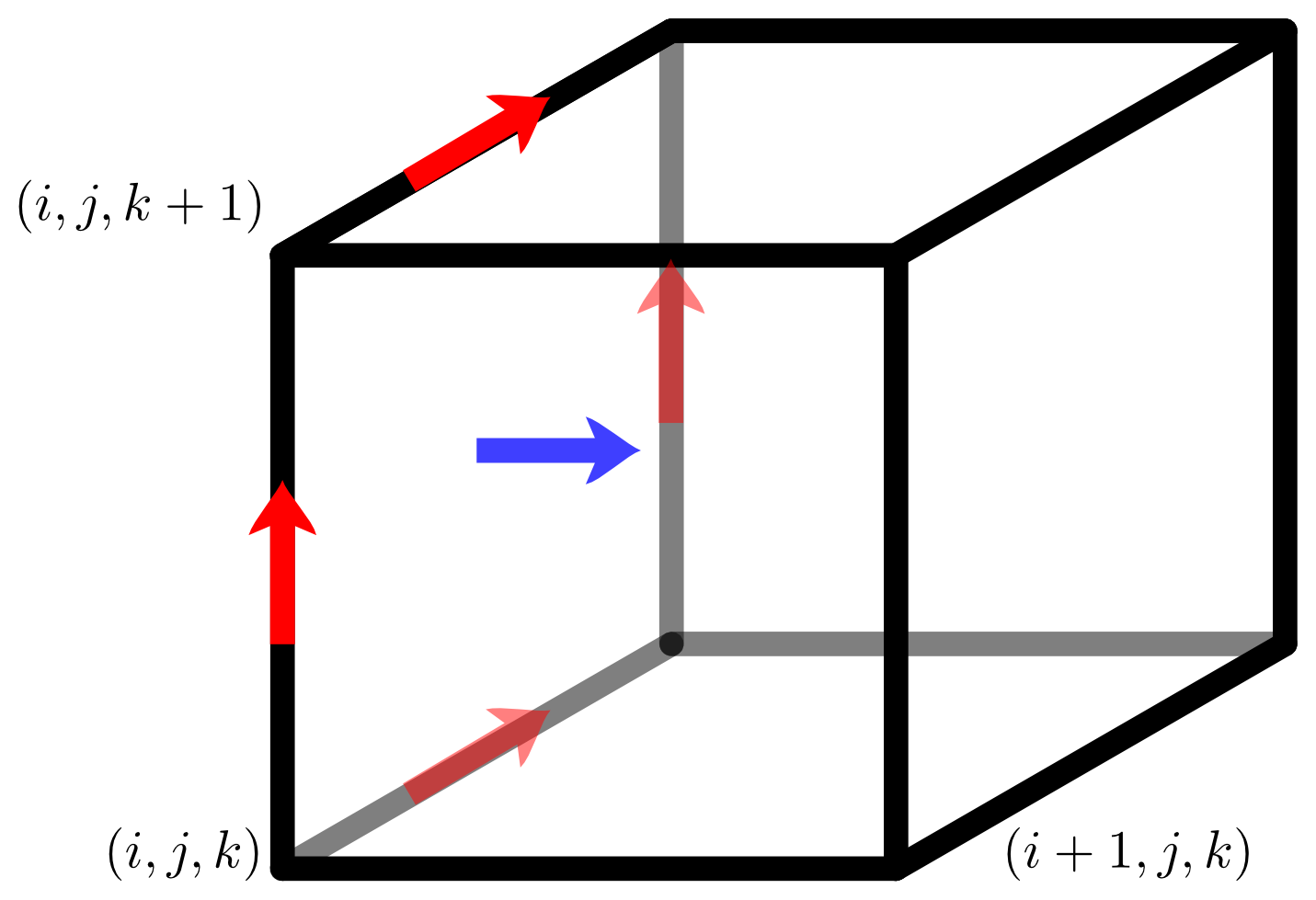The code of course implements the standard algorithm by Yee, 1966 that also drives the grid layout for the field quantities.
In this algorithm the curl of the electric field that is necessary to update B_x is computed from the four components of E_y and E_z that surround B_x in the staggered grid. The algorithm is straight forward and guarantees that the magnetic field does not gain a non-physcial divergence.
This algorithm is 2nd order accurate in dx, but is somewhat anisotropic and exhibits significant numerical dispersion along the coordinate axes.

#### 4th Order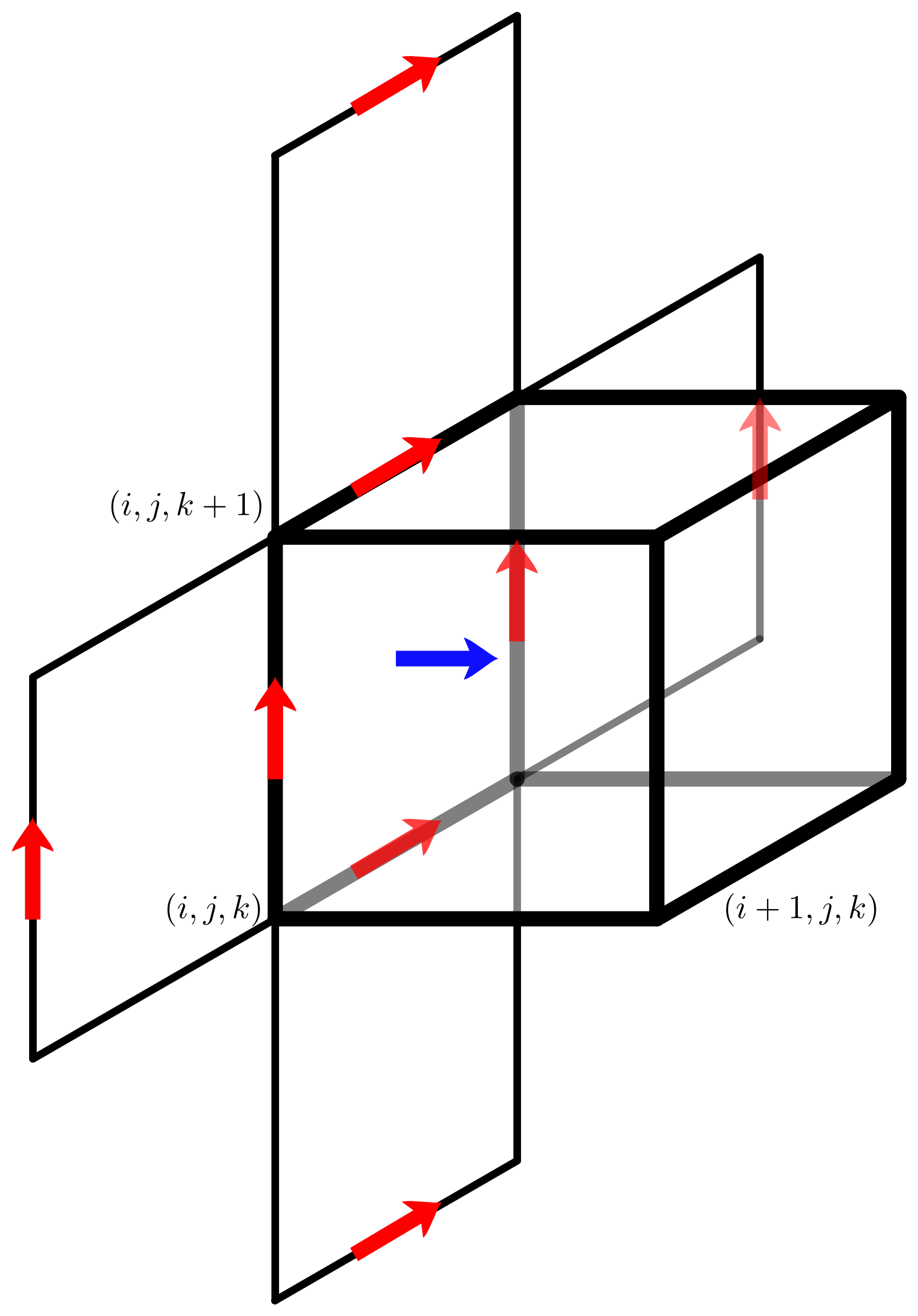In an attempt to improve the field solver a (naive) solver that is 4th order accurate was implemented. This solver uses additional grid location of E_y and E_z to compute curl(E) that enters the update of B_x. The big advantage is that it requires very limited modification of the code as the same grid layout can be used. The major downside is that the higher order of convergence is not visible at typical cell spacings and that smaller cell spacings where the faster convergence would be beneficial are typically prohibitively expensive.

#### NSFD (CK, CK5)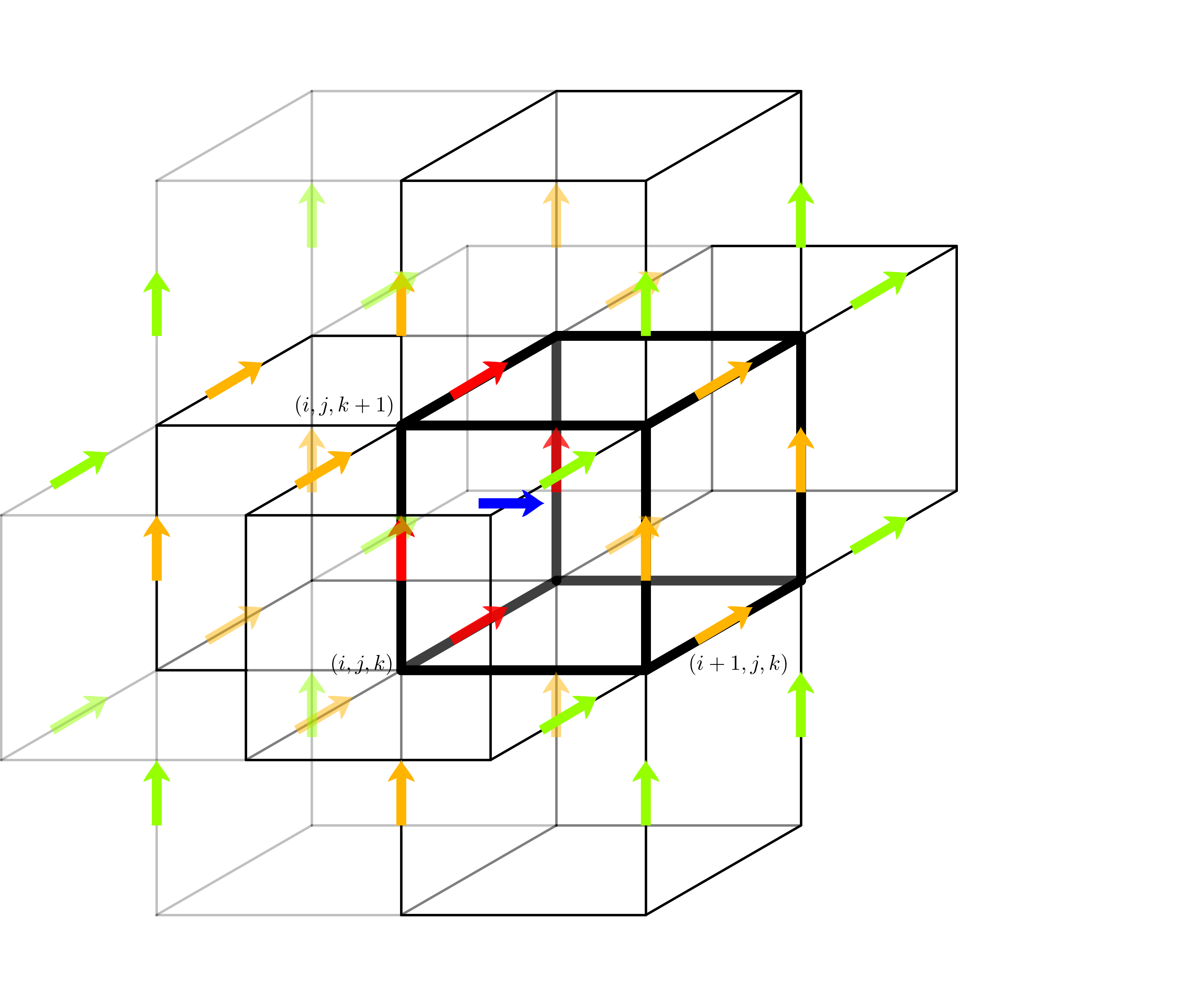The big improvement came with the realization that order of convergence is not as important as the error constant in front of the leading term. Here the non standard finite differences schemes proposed by Cole, 1997 and K�rkk�inen, 2006 excel. They use additional grid locations to compute the curl and optimize their relative weights to obtain numerical approximations of the curl operator that combine 2nd order accuracy with other desirable properties. A paper by Vay et al., 2011 discusses a number of potential parameters of such NSFD schemes. ACRONYM implements (in the nomenclature used there) the schemes CK and CK5, that offer maximally long time steps and maximally isotropic propagation, respectively.

#### M24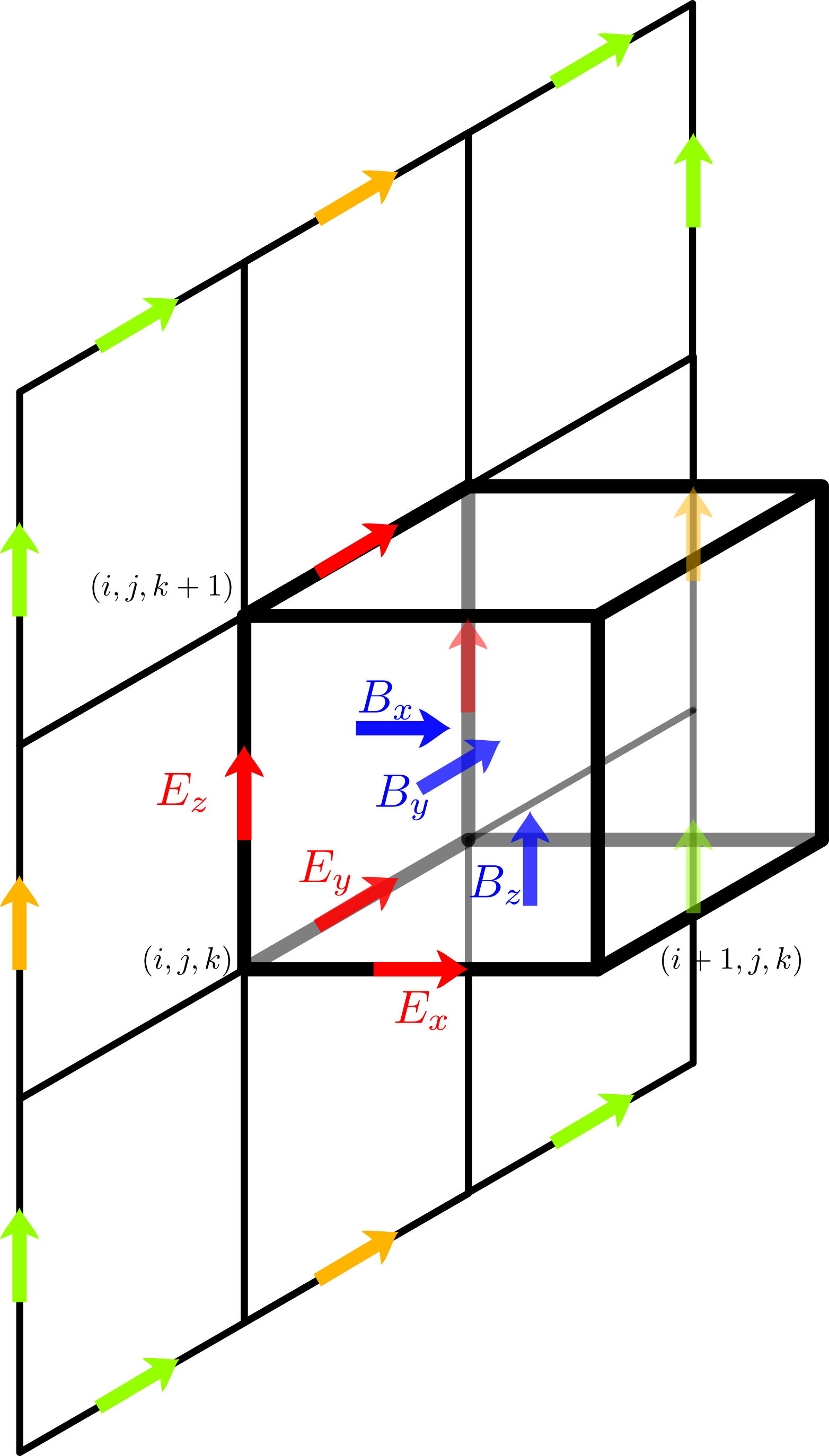In another modification of the Yee scheme Hadi, 1997 has proposed to use the grid locations used by the Yee scheme, the naive 4th order solvers and the grid location required to close the big outer loop. Again different relative weights are possible. Greenwood et al., 2004 have shown that a carefully chosen set of parameters can change the phase speed of electromagnetic waves suitably to remove grid Chernenkov effects. The scheme is not perfect and can therefore not remove grid Chernenkov radiation under all conditions, but the common case of axis parallel beams profits significantly. If Chernenkov radiation is still an issue the solver can be combined with Friedman filtering.

While the electrostatic plasma model neglects all effects O(v/c) and the electromagnetic model retains the finite speed of light, it is also possible to construct a plasma model that retains effects up to O(v^2/c^2), but ignores higher order effects. Interestingly enough there is a unique construction that does that which is equivalent to removing the displacement current and thus removing electromagnetic radiation. This results in longitudinal fields identical to the electrostatic plasma model, some transverse electric fields (but no light waves), and some corrections to the background magnetic field. Unfortunately the solver is quite complex and follows some unpublished work by Decyk. The biggest challenge is that the equation for the transverse electric field E_T needs the temporal change of the current density d j/dt as an input. Calculating this quantity from standard current deposition is too noisy. It is possible to rewrite the term, but at the cost of extra, numerically expensive, deposition steps.

## Boundary Conditions

The code in general allows for several boundary conditions, that can be different for different ends of the simulation domain. (Of course a periodic boundary at the lower end of the x axis needs to be connected to a periodic boundary at the upper end of the same axis.) The main challenge when creating boundary conditions is the need to have full consistency between the boundary formulation for the electromagnetic fields and the boundary conditions for the particles. Discrepancies lead to the formation of charged layers in the best case and violent instabilities in the worst case. Periodic boundary conditions are the easiest and most widely supported boundary conditions. Reflective boundary conditions are only supported by the electromagnetic plasma model. Absorbing boundary conditions are at least supported by electrostatic and electromagnetic solvers.

### Periodic Boundary Conditions

Periodic boundary conditions are straight forward and are actually handled internally in the same way as the interior inter-task boundaries, i.e. particles are sent to the new CPU and field values are copied into ghost zones. The wrap around is handled transparently by the geometry of the MPI Cartcom.

### Perfect Electric Conductor

Metal walls with perfect electric conductivity are modeled by setting the tangential electric field to zero. The current densities of particles close to the metal wall are adjusted using the current of an imaginary mirror charge on the other side of the wall. Since these boundary conditions are reflective to electromagnetic waves they are complemented on the particle side with specular reflection of incident particles. Unlike a real metal wall no charged particles are absorbed or reflected.

### Absorbing Boundary Conditions

The absorbing boundary conditions are implemented using a perfectly matched layer. Many different PML formulations exist. The current implementation uses an unsplit formulation. To improve absorption of low frequency waves a complex frequency shift approach is used, that requires a convolutional PML, i.e. the storage of auxiliary fields in the boundary region. On the particle side, outgoing particles are simply removed. The injection of new particles is handled by filling the ghost zone with new particles, performing one timestep inside the ghost zone and keeping the particles that enter the simulation domain.

## Output

Field quantities are written to self-describing HDF5 files in Pixie format using parallel HDF5 (i.e. one HDF5 file per simulation, for all CPUs and all timesteps). Particle data can be written to HDF5 tables or to binary files using MPI-IO.